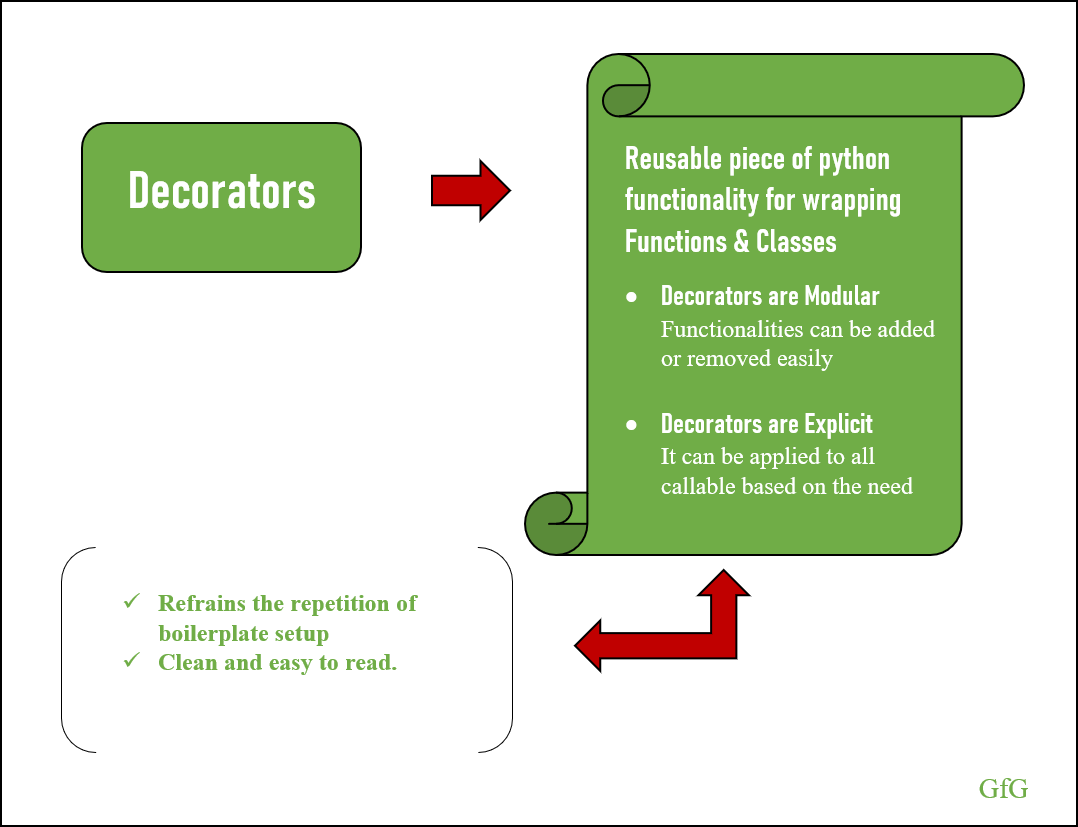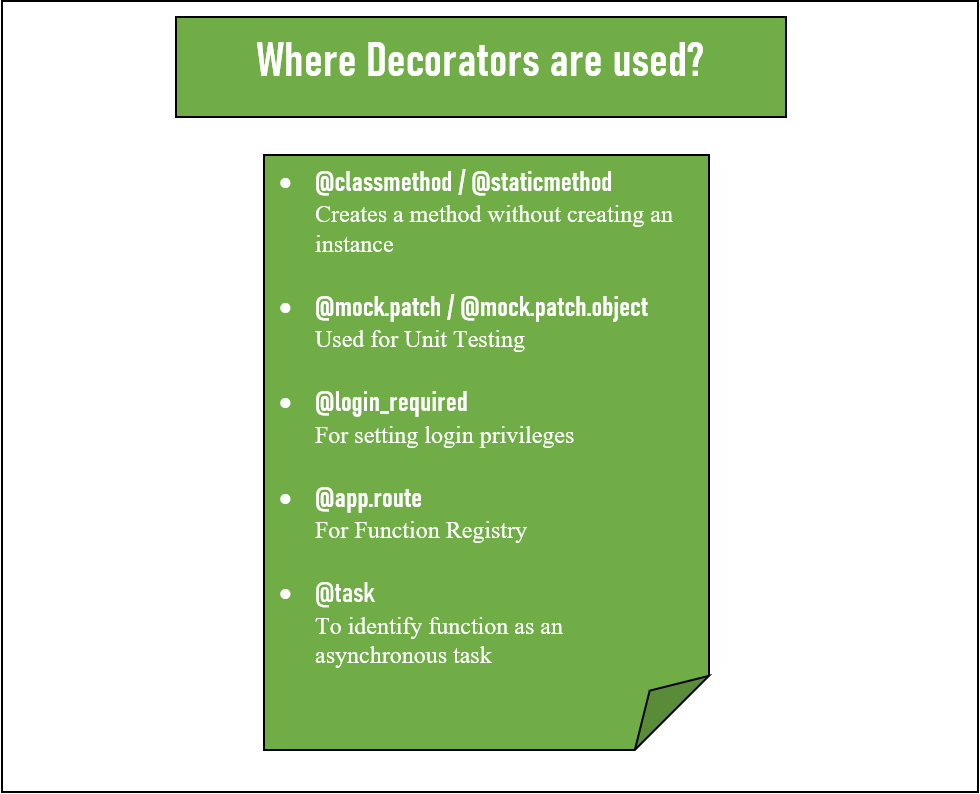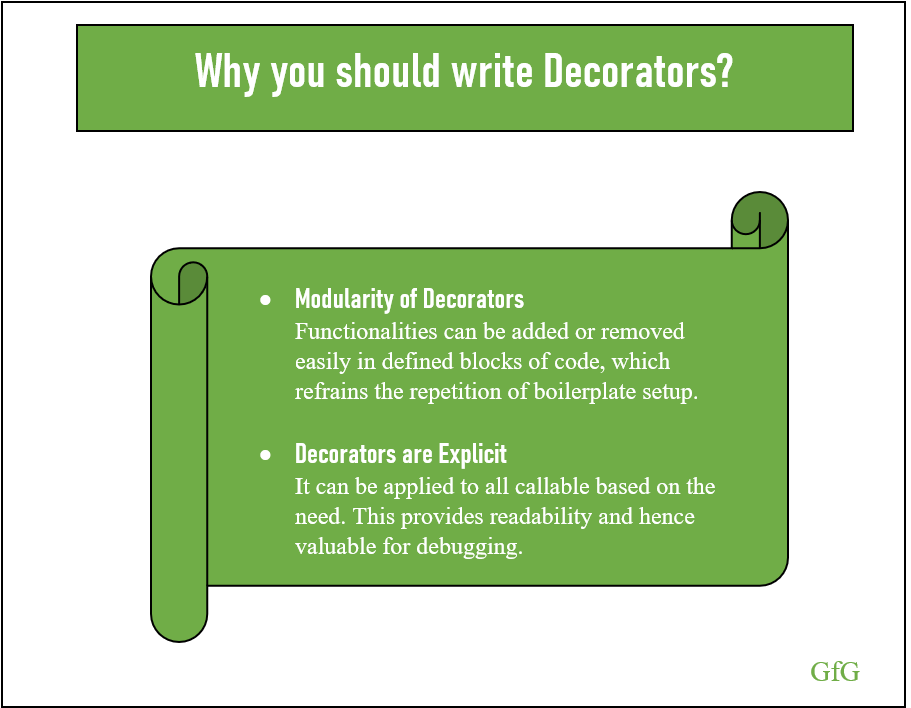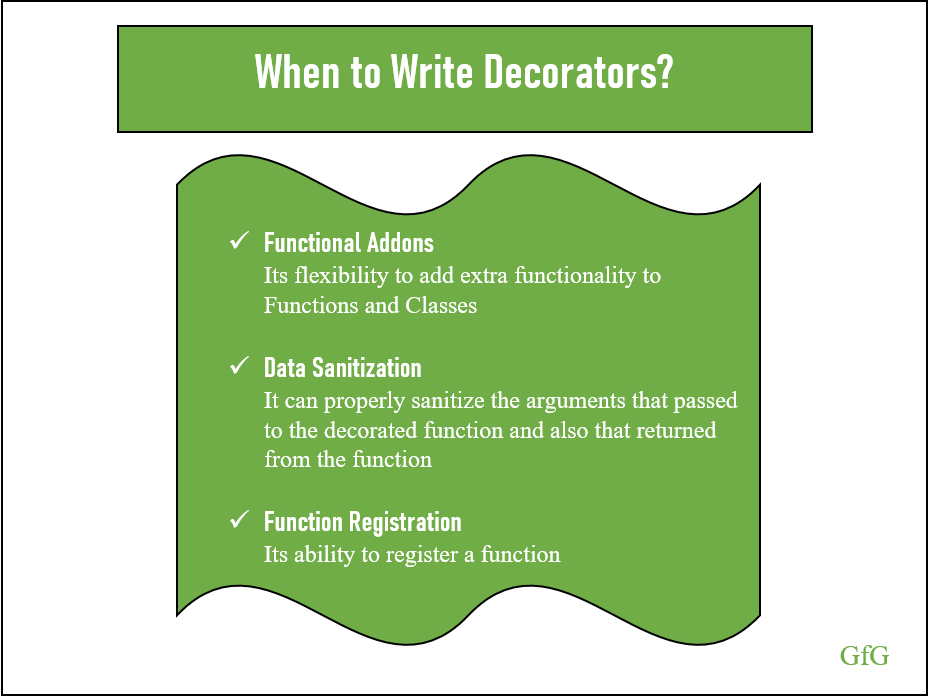# Python Decorators: A Complete Guide

A decorator is a design pattern tool in Python for wrapping code around functions or classes (defined blocks). This design pattern allows a programmer to add new functionality to existing functions or classes without modifying the existing structure. The section provides an overview of what decorators are, how to decorate functions and classes, and what problem can it solve.Decorators

## Understanding Decorators

A decorator is a function that takes another function as an argument, does some actions, and then returns the argument based on the actions performed. Since functions are first-class object in Python, they can be passed as arguments to another functions.
Hence we can say that a decorator is a callable that accepts and returns a callable.
Below code shows a simple decorator that add additional notes to the my_function docstring:

 `def` `decorated_docstring(function): ` `    ``function.__doc__ ``+``=` `'\n Hi George, I'``'m a simple Decorator.'` `    ``return` `function ` ` `  `def` `my_function(string1): ` `    ``"""Return the string."""` `    ``return` `string1 ` ` `  `def` `main(): ` `    ``myFunc ``=` `decorated_docstring(my_function) ` `    ``myFunc(``'Hai'``) ` `    ``help``(myFunc) ` ` `  `if` `__name__ ``=``=` `"__main__"``: ` `    ``main() `

Output:

```Help on function my_function in module __main__:

my_function(string1)
Return the string.
Hi George, Im a simple Decorator.
```

### Decorator Syntax

From the above explanation, you can understand how to decorate a function. But it is unacceptable to define a function, assign it to a variable, then reassign the decorated function to the same variable. Hence Python 2.5 introduced a syntax for decorating function by prepending an `@` character with the decorator name and adding directly above the function declaration. Below code shows how to use the syntax:

 `def` `reverse_decorator(function): ` ` `  `    ``def` `reverse_wrapper(): ` `        ``make_reverse ``=` `"".join(``reversed``(function())) ` `        ``return` `make_reverse ` ` `  `    ``return` `reverse_wrapper ` ` `  `@reverse_decorator` `def` `say_hi(): ` `    ``return` `'Hi George'` ` `  `def` `main(): ` `    ``print``(say_hi()) ` ` `  `if` `__name__ ``=``=` `"__main__"``: ` `    ``main() `

Output:

`egroeG iH`

Here we use @ character for decorating function.

 `@reverse_decorator` `def` `say_hi(): ` `    ``return` `'Hi George'`

The above code is syntactically equivalent to the following code `reverse_decorator(say_hi())`

In this case, the reverse_decorator function executes and creates the reference for revserse_wrapper. Let’s look into the below example for better understanding:

 `def` `reverse_decorator(function): ` `    ``print``(``'Inside reverse_decorator function'``) ` ` `  `    ``def` `reverse_wrapper(): ` `        ``print``(``'Inside reverse_wrapper function'``) ` `        ``return` `'Return reverse_wrapper function'` ` `  `    ``return` `reverse_wrapper ` ` `  ` `  `@reverse_decorator` `def` `say_hi(): ` `    ``return` `'Inside say_hi'`

Output:

`Inside reverse_decorator function`

Here the reverse_decorator does not execute the function reverse_wrapper instead it creates the reference and returns it when the callable invokes the function.

 `def` `reverse_decorator(function): ` `    ``print``(``'Inside reverse_decorator function'``) ` `   `  `    ``def` `reverse_wrapper(): ` `        ``print``(``'Inside reverse_wrapper function'``) ` `        ``return` `'reverse_wrapper'` ` `  `    ``return` `reverse_wrapper ` ` `  ` `  `@reverse_decorator` `def` `say_hi(): ` `    ``return` `'Inside say_hi'` ` `  `     `  `def` `main(): ` `    ``print``(``'Inside main()'``) ` `    ``print``(say_hi) ` `    ``print``('');  ` `    ``print``(say_hi()) ``# main executes the reference ` ` `  `main() `

Output:

Inside reverse_decorator function
Inside main()
<function reverse_decorator..reverse_wrapper at 0x0000015762A16708>

Inside reverse_wrapper function
reverse_wrapper

### Single Function and Multiple Decorator

Now you are clear about how to use the @syntax to decorate a function. Another cool stuff is that we can use multiple decorators on a single function. One important thing to note here is the order of the decorator applied is important, which is applied from bottom to top. Let’s look into multiple decorators.

 `def` `reverse_decorator(function): ` ` `  `    ``def` `reverse_wrapper(): ` `        ``make_reverse ``=` `"".join(``reversed``(function())) ` `        ``return` `make_reverse ` ` `  `    ``return` `reverse_wrapper ` ` `  `def` `uppercase_decorator(function): ` ` `  `    ``def` `uppercase_wrapper(): ` `        ``var_uppercase ``=` `function().upper() ` `        ``return` `var_uppercase ` ` `  `    ``return` `uppercase_wrapper ` ` `  `@uppercase_decorator` `@reverse_decorator` `def` `say_hi(): ` `    ``return` `'hi george'` ` `  `def` `main(): ` `    ``print``(say_hi()) ` ` `  `if` `__name__ ``=``=` `"__main__"``: ` `    ``main() `

Output:

`EGROEG IH`

Here the string is reversed first and foremost, and secondarily it converts to uppercase.

 `@uppercase_decorator` `@reverse_decorator` `def` `say_hi(): ` `    ``return` `'hi george'`

The above code is syntactically equivalent to
`uppercase_decorator(reverse_decorator(say_hi()))`

### Arguments in Decorator Function

So far, you have seen decorators without any arguments. In certain cases, it’s necessary to pass arguments to decorate the method accordingly.

 `def` `decorator_arguments(function): ` `    ``def` `wrapper_arguments(arg1, arg2): ` `        ``print``(``"Arguments accepted are: {0}, {1}"``.``format``(arg1, arg2)) ` `        ``function(arg1, arg2)  ``# calls the function hobbies ` ` `  `    ``return` `wrapper_arguments ` ` `  `@decorator_arguments` `def` `hobbies(hobby_one, hobby_two): ` `    ``print``(``"My Hobbies are {0} and {1}"``.``format``(hobby_one, hobby_two)) ` ` `  `def` `main(): ` `    ``hobbies(``"Travelling"``, ``"Reading"``) ` ` `  `if` `__name__ ``=``=` `"__main__"``: ` `    ``main() `

Output:

My Hobbies are Travelling and Reading

In the above case, the decorator will not take any arguments instead it is passed to the wrapper function by the callable. The below code provides more clarity in the process of passing the arguments using reference.

 `def` `decorator_arguments(function): ` ` `  `    ``def` `wrapper_arguments(arg1, arg2): ` `        ``print``(``" Arguments accepted are: {0}, {1}"``.``format``(arg1, arg2)) ` ` `  `    ``return` `wrapper_arguments ` ` `  `@decorator_arguments` `def` `hobbies(hobby_one, hobby_two): ` `    ``print``(``"My Hobbies are {0} and {1}"``.``format``(hobby_one, hobby_two)) ` ` `  `def` `main(): ` ` `  `    ``# the reference of wrapper_arguments ` `    ``# is returned ` `    ``hob ``=` `hobbies ` `    ``print``(hob) ` ` `  `    ``# passing the arguments to the  ` `    ``# wrapper_arguments ` `    ``hob(``'Travelling'``, ``'Reading'``) ` ` `  `main() `

Output

<function decorator_arguments..wrapper_arguments at 0x7f100315e158>

### Where Decorators are Used?The standard library provides many modules that include decorators and many Python tools and frameworks that make use of decorators. Few examples are:

• You can use @classmethod or @staticmethod decorator for creating a method without creating an instance
• The mock module permits the use of @mock.patch or @mock.patch.object as a decorator which is used for unit testing.
• Common tools such as Django (uses @login_required) for setting login privileges and Flask (uses @app.route) for function registry uses decorators.
• To identify a function as an asynchronous task Celery uses @task decorator.

### Why you should write DecoratorsTwo important reasons to write this reusable piece of Python functionality are when written properly the decorators are modular and explicit.

• Modularity of Decorators
• Decorators are Explicit

#### Modularity of Decorators

Using decorators’ programmers can add or remove functionalities easily in defined blocks of code, which refrains the repetition of boilerplate setup.

#### Decorators are explicit

Programmers can apply Decorators to all callable based on the need. This ensures readability and provides clean code.

### Decorator UseCasesHere we will discuss a few decorator use-cases which help you to understand When to write Decorators.

2. Data Sanitization
3. Function Registration

The first and foremost reason to write a decorator is its flexibility to add extra functionality to the defined blocks of code(functions and classes).

#### Data Sanitization

With proper data sanitization methods, you can remove or destroy the data that is stored on memory and make it impossible to recover it. For example, you may use the facility of cache to avoid running the same function or you may use methods to validate the user login credentials and so on, which points to the importance of data sanitization. A decorator can properly sanitize the arguments that passed to the decorated function and also sanitize the data returned from the function.

#### Function Registration

Another point to write a decorator is to register a function. Here decorator allows two or more subsystems to communicate without having much information about each other.

## Decorating Classes

The above section shows how decorators help in decorating functions. We have seen that decorators are callable that accepts and returns a callable. Since classes are callable,  decorators are also used to decorate classes. Some of the uses of decorating classes are:

• Addition or enhancement of attributes
• Attribute interaction
• Alter the API of a class (alter the way of declaring classes and its instance use)

Let’s go through how to decorate a function using class.

 `class` `MyDecorator: ` ` `  `    ``def` `__init__(``self``, function): ` `        ``self``.function ``=` `function ` ` `  `    ``def` `__call__(``self``): ` `        ``print``(``"Inside Function Call"``) ` `        ``self``.function() ` ` `  `@MyDecorator` `def` `function(): ` `    ``print``(``"GeeksforGeeks"``) ` ` `  `def` `main(): ` `    ``function() ` ` `  `if` `__name__ ``=``=` `"__main__"``: ` `    ``main() `

Output:

```Inside Function Call
GeeksforGeeks
```

Let’s look into an another example. Below code, sort the instance based on their creation time. Here we require three additional attributes- the instantiation timestamp, __lt__ and __gt__ methods.

 `import` `functools ` `import` `time ` ` `  `def` `sort_by_creation_time(cl): ` `    ``org_init ``=` `cl.__init__ ` ` `  `    ``# Enhance the class to store the creation ` `    ``# time based on the instantiation. ` `    ``@functools``.wraps(org_init) ` `    ``def` `new_init(``self``, ``*``args, ``*``*``kwargs): ` `        ``org_init(``self``, ``*``args, ``*``*``kwargs) ` `        ``self``._created ``=` `time.time() ` ` `  `    ``# __lt__ and __gt__ methods return true or false  ` `    ``# based on the creation time. ` `    ``cl.__init__ ``=` `new_init ` `    ``cl.__lt ``=` `lambda` `self``, other: ``self``._created < other._created ` `    ``cl.__gt__ ``=` `lambda` `self``, other: ``self``._created > other._created ` `    ``return` `cl ` ` `  `@sort_by_creation_time` `class` `Sort(``object``): ` `    ``def` `__init__(``self``, identifier): ` `        ``self``.identifier ``=` `identifier ` ` `  `    ``def` `__repr__(``self``): ` `        ``return` `self``.identifier ` ` `  ` `  `def` `main(): ` ` `  `    ``first ``=` `Sort(``'Python'``) ` `    ``second ``=` `Sort(``'Data Analysis'``) ` `    ``third ``=` `Sort(``'Machine Learning'``) ` ` `  `    ``sortables ``=` `[third, first, second] ` `    ``print``(``sorted``(sortables)) ` ` `  `if` `__name__ ``=``=` `"__main__"``: ` `    ``main() `

Output

```[Python, Data Analysis, Machine Learning]
```

`new_init`, its primary responsibility is to run the wrapped function and also add an extra functionality to the wrapped function. The `@functools.wraps(org_init)` update the wrapper function to reflect the look of wrapped function. Check functools for detail understanding.

 `@functools``.wraps(org_init) ` `def` `new_init(``self``, ``*``args, ``*``*``kwargs): `   `    ``# calls the init method of class ` `    ``org_init(``self``, ``*``args, ``*``*``kwargs) `   `    ``# add an extra attribute and store  ` `    ``# creation time   ` `    ``self``._created ``=` `time.time()  `   `# reference of new_init is assigned to ` `# __init__ method and executes when ` `# callable creates the class object. ` `cl.__init__ ``=` `new_init   `

## Decorate a Function and return a Class

Sometimes it’s necessary to decorate a function and return a class. Say, for example, advanced cases developers can subclass a class in an API. It can also avoid an increase in boilerplate code.

 `class` `Addition(``object``): ` ` `  `    ``def` `__call__(``self``, ``*``args, ``*``*``kwargs): ` `        ``return` `self``.run(``*``args, ``*``*``kwargs) ` ` `  `    ``def` `run(``self``, ``*``args, ``*``*``kwargs): ` `        ``raise` `NotImplementedError(``'Subclass must implement `run`.'``) ` `         `  `    ``def` `identity(``self``): ` `        ``return` `'Hi George, I'``'m here to help you with addition !'` ` `  `def` `addition(decorated): ` `    ``class` `AddSubclass(Addition): ` `        ``def` `run(``self``, ``*``args, ``*``*``kwargs): ` ` `  `            ``if` `args: ` `                ``add ``=` `0` `                ``for` `arg ``in` `args: ` `                    ``add ``=` `arg ``+` `add   ``# add arguments  ` `                ``return` `add ` `                 `  `            ``else``: ` `                ``# func is called if there are no arguments passed. ` `                ``return` `decorated(``*``args, ``*``*``kwargs)  ` `                                                     `  ` `  `    ``return` `AddSubclass() ` ` `  `@addition` `def` `func(``*``args): ` `    ``return` `1` `+` `1` ` `  `print``(func.identity()) ` `print``(func()) ` `print``(func(``2``, ``2``)) ` `print``(func(``2``, ``1``, ``4``)) `

Output

```Hi George, Im here to help you with addition!
2
4
7
```

Here the decorator creates a subclass of Addition and returns the instance of the subclass rather than reference (`return AddSubclass()`).

 `def` `addition(decorated): ` `    ``class` `AddSubclass(Addition): ` `        ``def` `run(``self``, ``*``args, ``*``*``kwargs): ` ` `  `            ``if` `args: ` `                ``add ``=` `0` `                ``for` `arg ``in` `args: ` `                    ``add ``=` `arg ``+` `add ` `                ``return` `add ` `                 `  `            ``else``: ` `                ``return` `decorated(``*``args, ``*``*``kwargs) ` ` `  `    ``return` `AddSubclass() `

And the __call__ method in the class `Addition` means that its instance is callable. Hence the instance of subclass `AddSubclass()` can run the task and as a result the programmer can avoid calling the run method as `func().run()`.

## Summary

Decorators are an excellent tool for wrapping code around functions and classes, which provides an efficient way to use boilerplate code and also contributes to readability. Even though they are modular and explicit, there are drawbacks to this tool. Since it’s a tool for wrapping code around defined blocks, debugging becomes more challenging. And also, poorly written decorators may result in an error.
After all, a well-written decorator can be treated as an efficient reusable piece of Python functionality for wrapping defined blocks of code.

My Personal Notes arrow_drop_upCheck out this Author's contributed articles.

If you like GeeksforGeeks and would like to contribute, you can also write an article using contribute.geeksforgeeks.org or mail your article to contribute@geeksforgeeks.org. See your article appearing on the GeeksforGeeks main page and help other Geeks.

Please Improve this article if you find anything incorrect by clicking on the "Improve Article" button below.

Article Tags :

Be the First to upvote.

Please write to us at contribute@geeksforgeeks.org to report any issue with the above content.